# 一文学懂动态规划

## 前言

$$f(n) = f(n-1)+f(n-2)$$

class Solution {
public int fib(int N) {
if (N == 0) {
return 0;
} else if (N == 1) {
return 1;
}
return fib(N-1) + fib(N-2);
}
}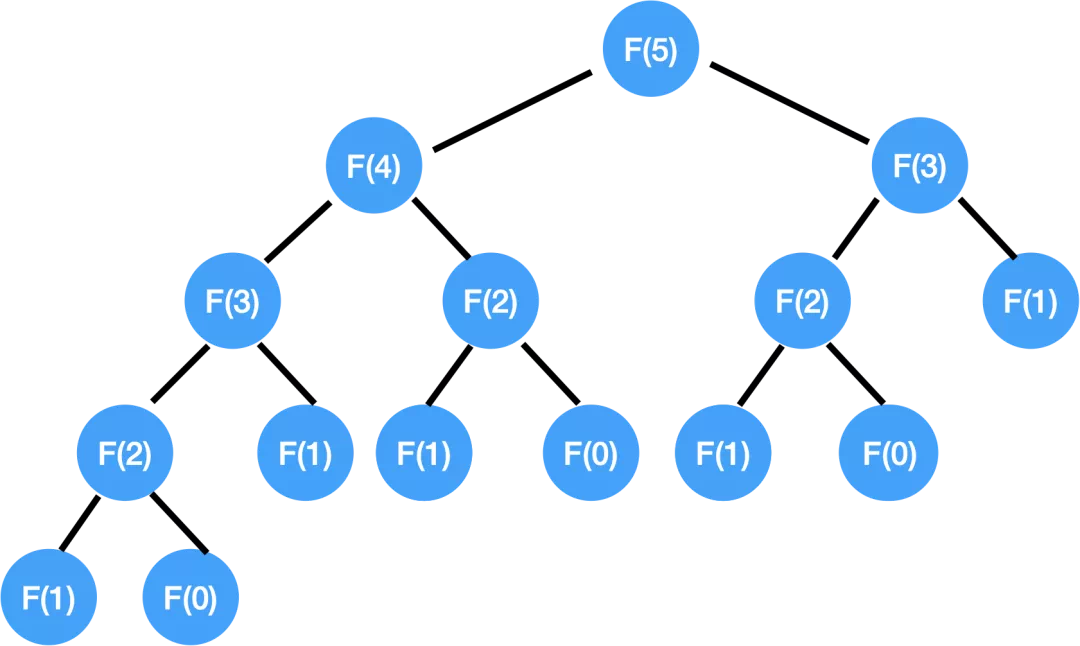$$O(2^n)$$

class Solution {
public int fib(int N) {
int a = 0;
int b = 1;
if(N == 0) {
return a;
}
if(N == 1) {
return b;
}
for(int i = 2; i <= N; i++) {
int tmp = a + b;
a = b;
b = tmp;
}
return b;
}
}

## 思路分析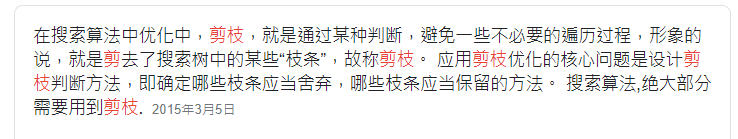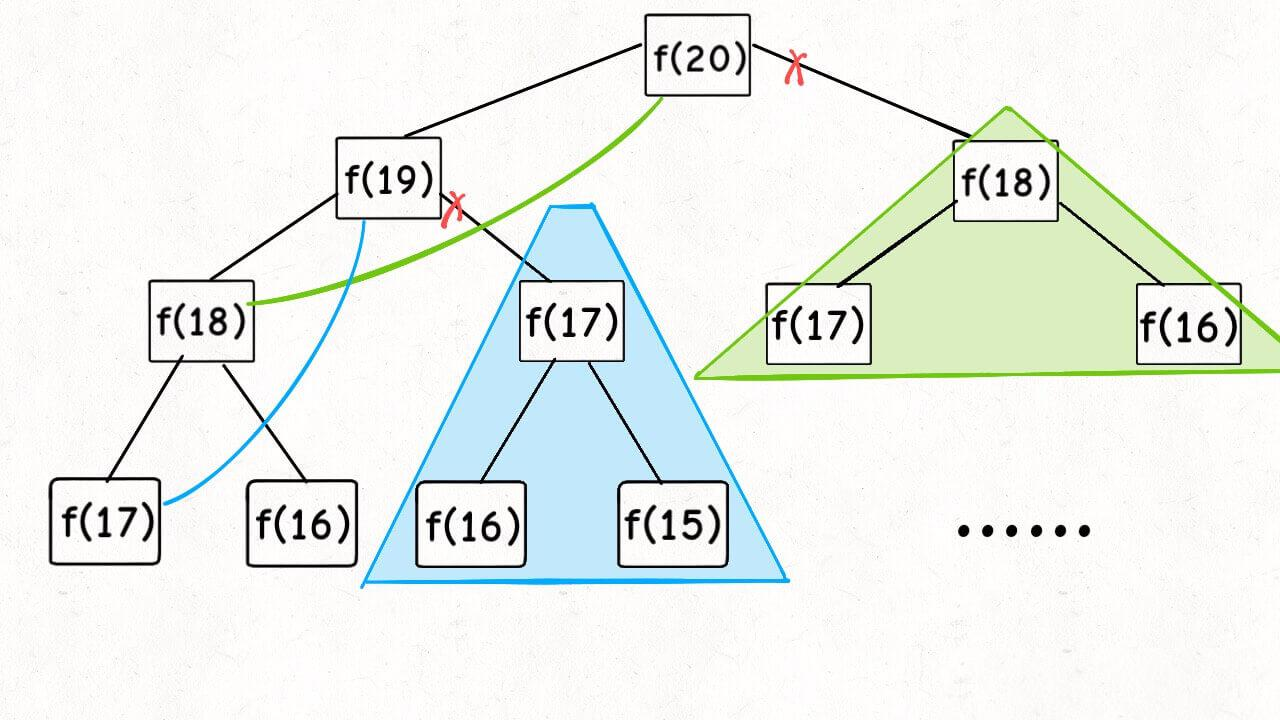## 动态规划核心

1. 重叠子问题
2. 最优子结构

1. 状态转移方程

### 1. 重叠子问题

$$f(n) = f(n-1)+f(n-2)$$

### 2. 最优子结构

#### 零钱兑换

输入：coins = [1, 2, 5], amount = 11

输入：coins = , amount = 3

### 1. 重叠子问题

1. 我们可以先凑出 11 块（此时我们已经用了一枚 11 块的硬币，硬币数+1），然后再凑出 15 - 11 = 4 块。

假设 f(n)表示凑出 n 元所需要的最少硬币数， 那么这样我们凑出 15 块所需要的硬币总数为 f(15) = f(4) + 1

2. 我们也可以先凑出 5 块，然后再凑出 15 - 5 = 10 块。

f(15) = f(10) + 1

3. 我们也可以先凑出 1 块，然后再凑出 15 - 1 = 14 块。

f(15) = f(14) + 1

### 2.最优子结构

f(n) 只与 f(n-1)f(n-5)f(n-11) 的值相关。

### 3.状态转移方程

$$f(n) = min[f(n -1),f(n -5),f(n - 11)] + 1$$

$$f(n) = f(n-1)+f(n-2)$$

• 将自顶向下的递归，变成了自底向上的 for loop。

• 将每次计算出来的节点值予以保存。

Fibonacci 例子中，我们用 notes[n] 来表示输入 n 时的返回值答案，这里我们统一用 dp table.

int[] dp = new int[amount+1];

dp = 0;

// Fibonacci
public int fib(int N) {
if (N == 0) {
return 0;
} else if (N == 1) {
return 1;
}
return fib(N-1) + fib(N-2);
}

$$f(n) = min[f(n -1),f(n -5),f(n - 11)] + 1$$

coins = {1,2,5,7,10} amount = 14

int[] dp = int;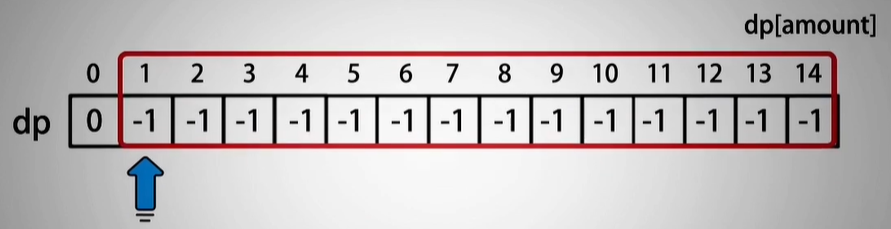for(int i = 1;i <= amount;i++){

...

}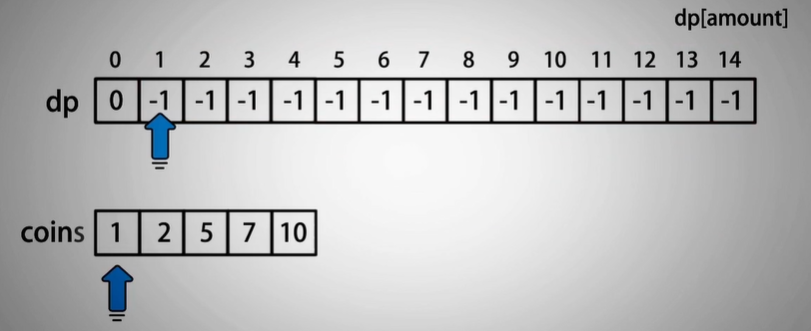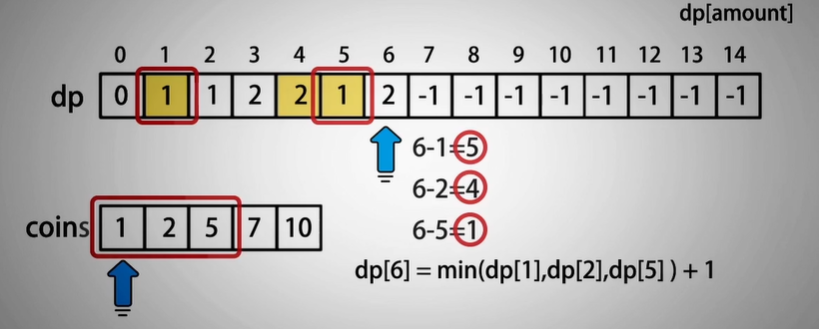6 - 1 = 5 dp = 1

6 - 2 = 4 dp = 2

6 - 5 = 1 dp = 1

dp = min(dp,dp,dp) + 1

//来一个整型最大数，保证其它数第一次和这个数比较时都比这个数小
//变量名叫min，这是对应外层i循环，即:求每个dp[i]的最优解
int min = Integer.MAX_VALUE;
for(int j = 0;j < coins.length;j++){
//所有小于等于i的面值coins[j]，并且最优解小于默认最大值
if(coins[j] <= i && dp[i - coins[j]] < min){
min = dp[i - coins[j]] + 1;//更新dp
}
}
dp[i] = min;

class Solution {
public int coinChange(int[] coins, int amount) {
if(coins.length == 0) return -1;
int[] dp = new int[amount + 1];
dp = 0;
for(int i = 1;i <= amount;i++){
int min = Integer.MAX_VALUE;
for(int j = 0;j < coins.length;j++){
if(coins[j] <= i && dp[i - coins[j]] < min){
min = dp[i - coins[j]] + 1;
}
}
dp[i] = min;
}
return dp[amount] == Integer.MAX_VALUE ? -1 : dp[amount];
}
}

## 总结

1. 首先分析这个问题符不符合动态规划最重要的前两个性质（重叠子问题，最优子结构）；
2. 如果满足前两个性质，那么我们尝试写出状态转移方程，也即递归式
3. 优化：将自顶向下的递归式（_函数调用_）改为自底向上动态规划（_for loop_）

11-229706-28184
09-211928
02-0585
11-132万+
04-0110
02-2029
06-271619
10-09152
08-23134
09-07107
12-061290
04-1323
12-2346
03-1040
04-0411
08-2751
11-241596
©️2020 CSDN 皮肤主题: Age of Ai 设计师:meimeiellie